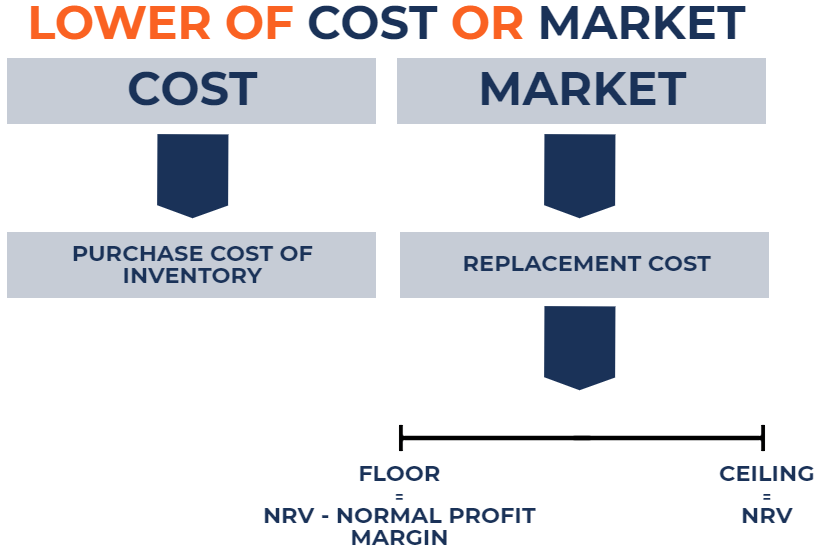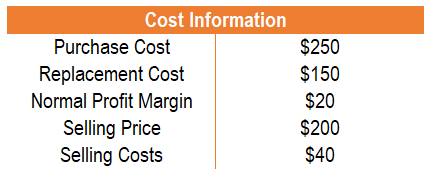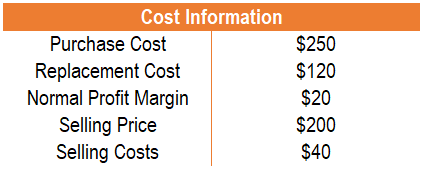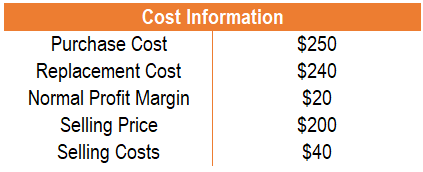# Lower of Cost or Market (LCM)

An inventory valuation method required for companies that follow U.S. GAAP

## What is Lower of Cost or Market (LCM)?

Lower of cost or market (LCM) is an inventory valuation method required for companies that follow U.S. GAAP. In the lower of cost or market inventory valuation method, as the name implies, inventory is valued at the lower of cost or market.### Quick Summary:

• Lower of cost or market (LCM) is an inventory valuation method required for companies that follow U.S. GAAP.
• Cost refers to the purchase cost of inventory, and market cost refers to the replacement cost of inventory.
• The replacement cost cannot exceed the net realizable value or be lower than the net realizable value less a normal profit margin.

### Rationale Behind Lower of Cost or Market (LCM)

When inventory is purchased by a company, it sits on the balance sheet at cost. However, with the passage of time, the value of the inventory may depreciate or appreciate. To increase the reliability of financial statements, the changing value of inventory, to an extent, must be accounted for.

For example, if a company purchased inventory at the cost of \$100,000 but the market value of the inventory is \$20,000, users of financial statements would want the lower value to be reflected in the books. If the inventory value was not reassessed to the appropriate value, it would overstate assets of the company and mislead users. However, as will be discussed below, the lower of cost or market inventory valuation method is not as simple as just comparing cost and market.

### Valuing Inventory at Lower of Cost or Market (LCM)

In the lower of cost or market inventory valuation method, the inventory of the company purchased at cost is compared against the market value of that inventory. The market value of inventory is essentially the replacement cost of that inventory. For example, if the replacement cost for an inventory of a company is \$100, that is the same as saying that the market value of that inventory is \$100. However, there are some caveats for replacement value:

1. The replacement cost cannot exceed the net realizable value (NRV).
2. The replacement cost cannot be lower than net realizable value less a normal profit margin.

Net realizable value is the sale price of the inventory minus any costs incurred to prepare the inventory for sale. A normal profit margin is the average spread between the cost and sale price of the inventory. Such caveats for replacement cost establish a floor and ceiling for replacement cost. It is illustrated as follows:Here are the steps to valuing inventory at the lower of cost or market:

1. First, determine the purchase cost of inventory.

2. Second, determine the replacement cost of inventory. It is the same as the market value of inventory.

3. Compare replacement cost to net realizable value and net realizable value minus a normal profit margin. If:

• Replacement cost > net realizable value, use net realizable value for replacement cost.
• Replacement cost < net realizable value minus a normal profit margin, use net realizable value minus a profit margin for replacement cost.
• Net realizable value minus a normal profit margin < replacement cost < net realizable value, use replacement cost.

4. Compare the cost of inventory to replacement cost. Lastly, if:

• Cost of inventory < replacement cost, a write-down is not necessary.
• Cost of inventory > replacement cost, write-down inventory to replacement cost.

To fully understand the concepts, a comprehensive example is prepared below.

### Examples of Lower of Cost or Market (LCM)

#### Example 1

ABC Company sells wallets. Cost information regarding the inventory of ABC Company is presented below:• The purchase cost: \$250
• The replacement cost: \$150
• The net realizable value: \$160 (\$200 – \$40)
• The net realizable value minus a normal profit margin: \$140 (\$160 – \$20).

In this example, replacement cost falls between net realizable value and net realizable value minus a normal profit margin. Therefore, the replacement cost used is \$150. Comparing the amount to the purchase cost of \$250, a \$100 write-down is necessary.

#### Example 2

ABC Company sells wallets. Cost information regarding the inventory of ABC Company is presented below:• The purchase cost: \$250
• The replacement cost: \$120
• The net realizable value: \$160 (\$200 – \$40)
• The net realizable value minus a normal profit margin: \$140 (\$160 – \$20)

In this example, replacement cost falls below the net realizable value minus a normal profit margin. Therefore, the replacement cost used is \$140. Comparing the amount to the purchase cost of \$250, a \$110 write-down is necessary.

#### Example 3

ABC Company sells wallets. Cost information regarding the inventory of ABC Company is presented below:• The purchase cost: \$250
• The replacement cost: \$240
• The net realizable value: \$160 (\$200 – \$40)
• The net realizable value minus a normal profit margin: \$140 (\$160 – \$20)

In this example, replacement cost is above net realizable value. Therefore, the replacement cost used is \$160. Comparing the amount to the purchase cost of \$250, a \$90 write-down is necessary.

### Recording Lower of Cost or Market

If the market cost is lower than cost, a write-down is necessary. The journal entry would be as follows:

 Loss from the decline in inventory value Dr. XX Inventory Cr. XX

The loss from the decline in inventory value would be reflected in the income statement and reduce net income. Inventory would be reflected in the balance sheet and reduce the value of inventory.

The journal entry for the three examples above would be:

#### Example 1

 Loss from the decline in inventory value Dr. 100 Inventory Cr. 100

#### Example 2

 Loss from the decline in inventory value Dr. 110 Inventory Cr. 110

#### Example 3

 Loss from the decline in inventory value Dr. 90 Inventory Cr. 90

### More Resources

CFI is the official provider of the global Financial Modeling & Valuation Analyst (FMVA)™ certification program, designed to help anyone become a world-class financial analyst. To keep advancing your career, the additional CFI resources below will be useful:

• IFRS vs. US GAAP
• Inventory Audit
• Market Valuation Approach
• T Accounts Guide